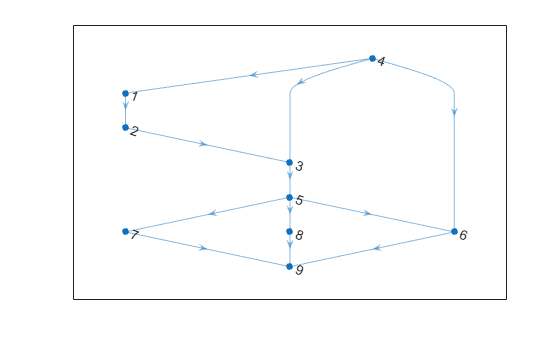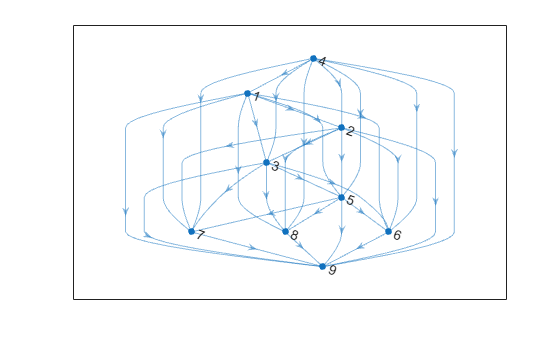# transclosure

Transitive closure

## Syntax

``H = transclosure(G)``

## Description

example

````H = transclosure(G)` returns the transitive closure of graph `G` as a new graph, `H`. The nodes in `H` are the same as those in `G`, but `H` has additional edges. If there is a path from node `i` to node `j` in `G`, then there is an edge between node `i` and node `j` in `H`. For multigraphs with multiple edges between the same two nodes, the output graph replaces these with a single edge.```

## Examples

collapse all

Create and plot a directed graph.

```G = digraph([1 2 3 4 4 4 5 5 5 6 7 8],[2 3 5 1 3 6 6 7 8 9 9 9]); plot(G)```Find the transitive closure of graph `G` and plot the resulting graph. `H` contains the same nodes as `G`, but has additional edges.

```H = transclosure(G); plot(H)```The transitive closure information in `H` can be used to answer reachability questions about the original graph, `G`.

Determine the nodes in `G` that can be reached from node 1. These nodes are the successors of node 1 in the transitive closure graph, `H`.

`N = successors(H,1)`
```N = 7×1 2 3 5 6 7 8 9 ```

Create and plot a directed graph.

```s = [1 1 2 2 3 4 4 5]; t = [2 4 3 4 5 5 6 6]; G = digraph(s,t); plot(G,'Layout','subspace')```Calculate the adjacency matrix of the transitive closure of `G`. The result is a reachability matrix, which has nonzero values to indicate which nodes are reachable from each node.

```D = transclosure(G); R = full(adjacency(D))```
```R = 6×6 0 1 1 1 1 1 0 0 1 1 1 1 0 0 0 0 1 1 0 0 0 0 1 1 0 0 0 0 0 1 0 0 0 0 0 0 ```

For example, to answer the question "Which nodes are reachable from node 3?", you can look at the third row in the matrix. That row indicates only nodes 5 and 6 are reachable from node 3:

`find(R(3,:))`
```ans = 1×2 5 6 ```

## Input Arguments

collapse all

Input graph, specified as a `digraph` object. Use `digraph` to create a directed graph object.

Example: `G = digraph([1 2],[2 3])`

## Output Arguments

collapse all

Transitive closure of `G`, returned as a `digraph` object. The table `G.Nodes` is copied to `H`, but any properties in `G.Edges` are dropped.

Use `successors(H,n)` to determine the nodes in `G` that are reachable from node `n`.

collapse all

### Transitive Closure

The transitive closure of a graph describes the paths between the nodes. If there is a path from node `i` to node `j` in a graph, then an edge exists between node `i` and node `j` in the transitive closure of that graph. Thus, for a given node in the graph, the transitive closure turns any reachable node into a direct successor (descendant) of that node.